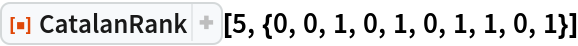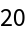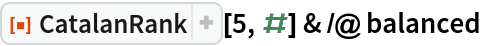#Function Repository Resource:

# CatalanRank

Find the rank of a totally balanced binary sequence

Contributed by: Ed Pegg Jr
 ResourceFunction["CatalanRank"][n,bin] returns the position of the given totally balanced binary sequence bin with n ones, in a particular enumeration of all such balanced sequences.

## Details and Options

A binary sequence is considered totally balanced if the number of zeros is at least as large as the number of ones as one progresses from left to right in a list of zeros and ones, and the total counts are equal (implying the first element must be zero and the last element one).
For n ones there are Cn totally balanced binary sequences, where Cn is the Catalan number.
The value returned is an index into a certain ordering of the set of all possible balanced sequences with n zeros and n ones, with indexing starting at 0.
The indexing scheme can be inverted using the resource function CatalanUnrank.
Brackets in a computer program must be balanced. One can think of a proper bracketing as having left brackets corresponding to zeros and right brackets to ones in a balanced binary sequence.

## Examples

### Basic Examples (2)

Find the rank of a given balanced binary sequence:

 In:=Out=Find all totally balanced binary sequences of length 10:

 In:=Out=Find the Catalan rank of each balanced sequence:

 In:=Out=